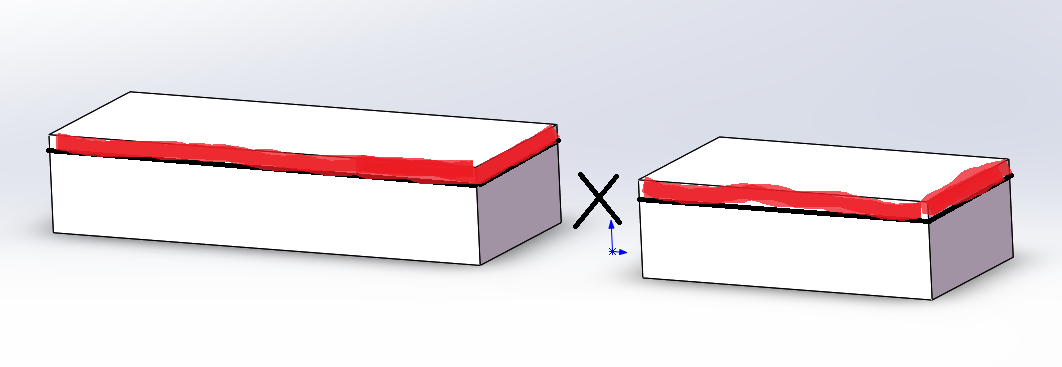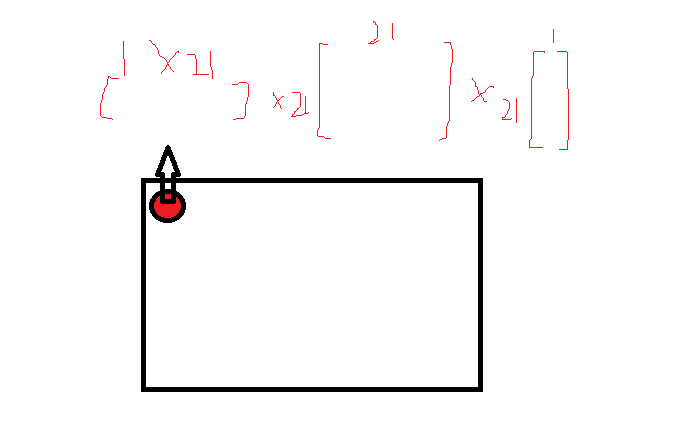# Matlab三维数组与二维矩阵相乘问题

P = A H ∗ ( U ∗ U H ) ∗ A P=A^{H} *(U*U^{H})*A1. 将A矩阵交换行列，变为21x1801x691
2. 使用reshape指令，将A平铺为21x(1801x691)的二维矩阵
3. 计算 ( U ∗ U H ) ∗ A (U*U^{H})*A
4. 计算结果再与 A H A^{H} 相乘，这里可以用bsxfun实现点对点的相乘

A=exp(GAMMA+PHI);%任意给定3维矩阵即可
A_1=permute(A,[3,2,1]);%交换行列
A_2=reshape(A_1,length(UU),length(incidence_angle)*length(distance));%平铺
U_times_A_1=UU*A_2;%相乘
U_times_A=reshape(U_times_A_1,length(UU),length(incidence_angle),length(distance));%重新组合成三维
U_times_A=permute(U_times_A,[3,2,1]);%重新交换行列
P_1=sum(bsxfun(@times,conj(A),U_times_A),3);


03-1627702-262068
05-061761
02-272245
12-041万+
07-251万+
02-19279
07-101万+
11-06955
08-30
10-10
04-111万+
12-223万+
07-207110
09-201万+点击重新获取扫码支付余额充值